If you’re preparing for the Medical College Admission Test (MCAT), you will want to consult the experts. These selections from Kaplan’s MCAT Question of the Day series can help you sharpen your skills as you prepare to begin your potential journey into medical training.

The questions below come from three of the four MCAT sections—biological and biochemical foundations of living systems; chemical and physical foundations of biological systems; and psychological, social, and biological foundations of behavior. A fourth section, critical analysis and reasoning skills (commonly referred to as CARS), is based largely on inference. Medicine can be a career that is both challenging and highly rewarding but figuring out a medical school’s prerequisites and navigating the application process can be a challenge unto itself. For students preparing for medical school, the AMA pre-med glossary guide has the answers to frequently asked questions.

Question: What is the IUPAC name for the compound shown below?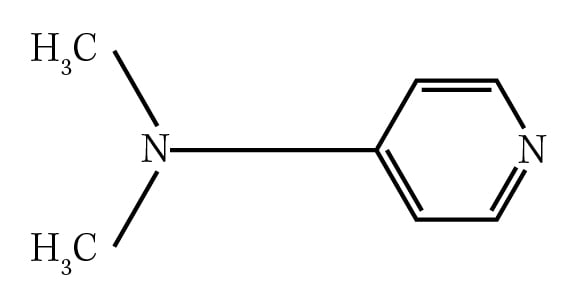A. 4-(N-Dimethylamino)pyridine

B. Dimethylaminopyridine

C. 4-(N,N-Dimethylamino)pyridine

D. N,N-Dimethylaminopyridine

Kaplan explains why: All groups (except hydrogen atoms) bonded to nitrogen need to be specified by the N- prefix, followed by the group. This prefix is repeated for each group. Choice A is incorrect because it fails to provide an N- prefix for each methyl substituent, whereas choice B is incorrect because it lacks both a numerical position and N- prefixes. Choice D is incorrect because the position of the amino substituent is not specified.

#### Related Coverage

March 2022: Kaplan MCAT stumpers put pre-meds to the test

Question: In the process of photosynthesis, carbon dioxide and water combine with energy to form glucose and oxygen, according to the following equation.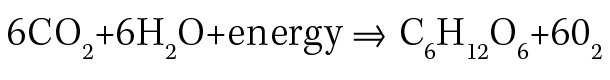What is the theoretical yield, in grams, of glucose if 30 grams of water are reacted with excess carbon dioxide and energy, in the balanced equation?

A. 300.1 grams glucose

B. 50.02 grams glucose

C. 30.03 grams glucose

D. 1,801 grams glucose

Kaplan explains why: This is a popular equation that you’ve probably all seen before. What’s missing from it are the coefficients! This is an unbalanced equation. In order to get anywhere with this problem, you have to balance it first.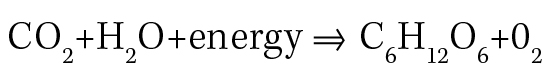The theoretical yield is the amount of product synthesized if the limiting reagent is completely used up. This question therefore asks how much glucose is produced if the limiting reagent is 30 grams of water. First, calculate the number of moles of water represented by 30 grams by dividing by its molecular weight (18.01 g/mol). You’ll have a lot of conversion factors, so wait until the end to multiply it all out.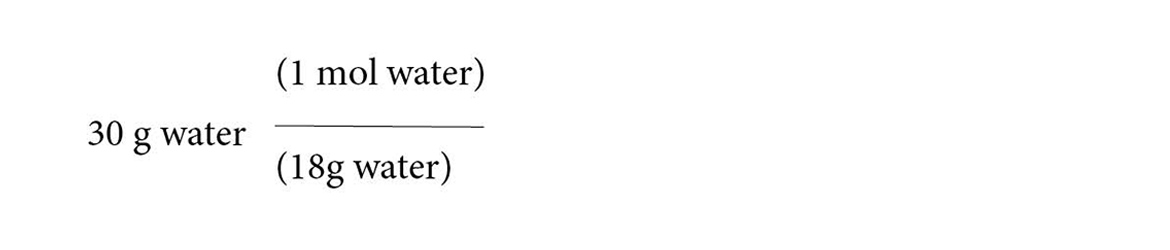Next, add conversion factors to find the equivalent number of moles of glucose: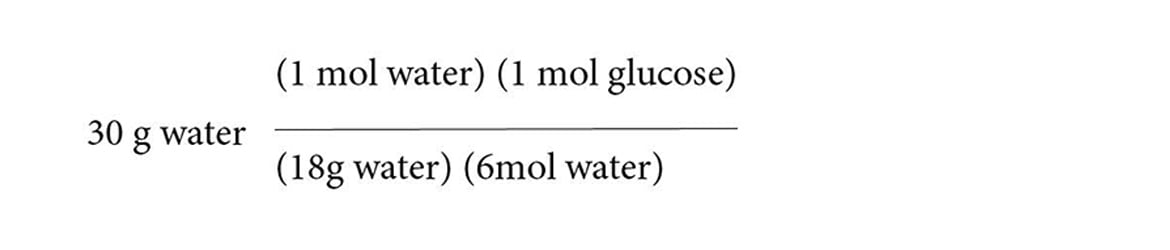Finally, convert moles of glucose into grams of glucose by multiplying by its molecular weight (180.2 g/mol glucose).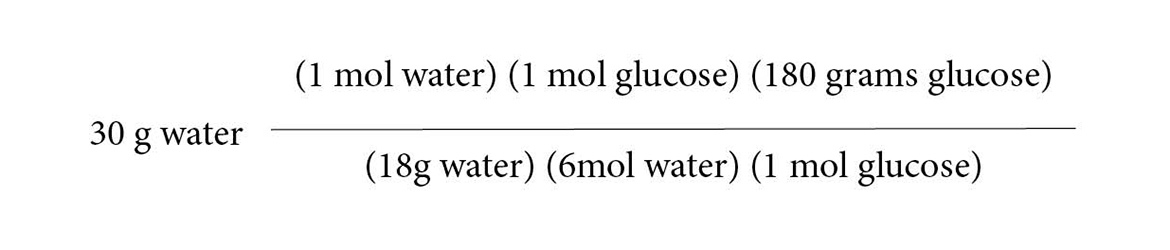After crossing out equal terms from the numerator and denominator, you are left with: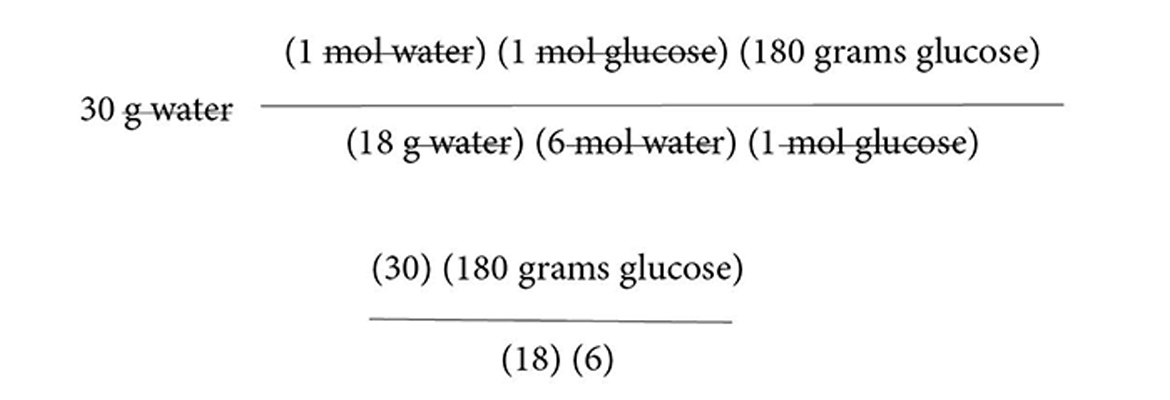Dividing 18 and 6 into our numerators will yield 50.02 grams of glucose.

#### Related Coverage

February 2022: Kaplan MCAT stumpers put pre-meds to the test

Question: Which of the following is an example of an implicit memory?

A. Noticing the feel of the ball as you shoot a free throw.

B. Knowing and reciting the steps to making an accurate free throw.

D. Shooting an accurate free throw without consciously recalling how.

Kaplan explains why: An implicit memory is a type of long-term memory that does not require conscious recall.  Typical examples would be skills we can perform automatically, such as riding a bike or touch typing. Choice D is a perfect match for this concept, and so is correct. Choices B and C are examples of the two main types of explicit memory, with knowing facts as in B representing semantic memory and C demonstrating episodic memory. Finally, choice A would be an example of sensory memory (haptic memory, specifically).

Explore Series
MCAT Stumpers

## RELATED CONTENT

Featured Stories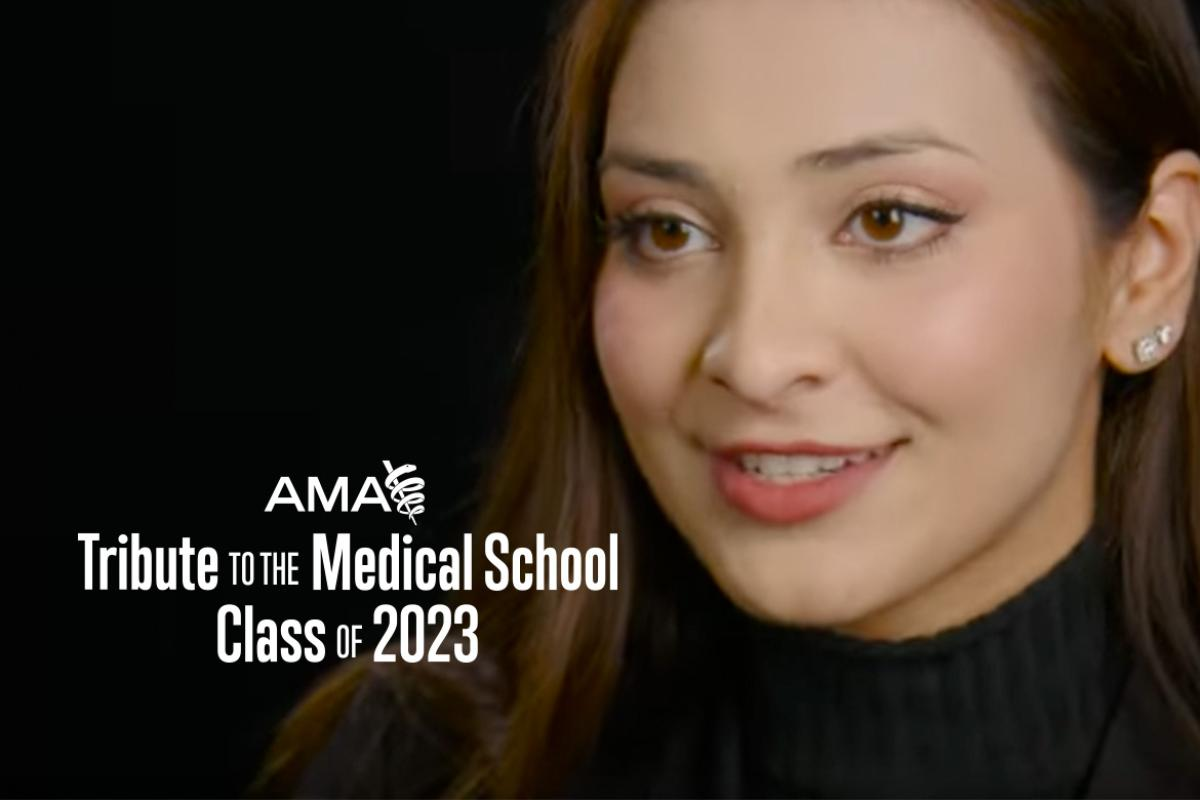### Huge names help AMA pay tribute to medical school class of 2023### Don’t expand scope of practice for already overworked pharmacists